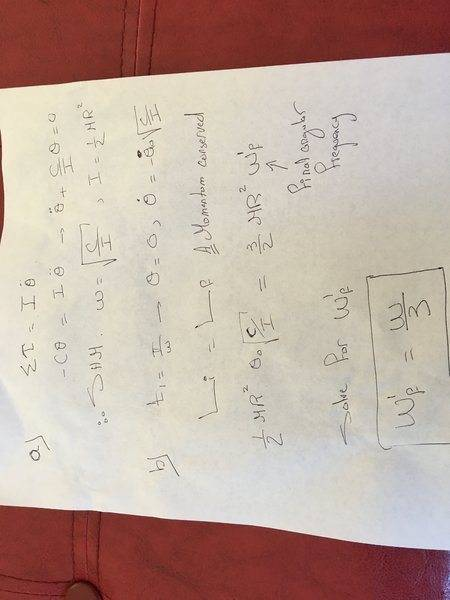# Kleppner Mechanics: Disk and coil spring

MARX

## Homework Statement

A solid disk of mass M and radius R is on a vertical shaft. The shaft is attached to a coil spring that exerts a linear restoring torque of magnitude Cθ, where θ is the angle measured from the static equilibrium position and C is a constant. Neglect the mass of the shaft and the spring, and assume the bearings to be frictionless.

(a) Show that the disk can undergo simple harmonic motion, and find the frequency of the motion.

(b) Suppose that the disk is moving according to θ = θ0 sin (ωt), where ω is the frequency found in part (a). At time t1 = π/ω, a ring of sticky putty of mass M and radius R is dropped concentrically on the disk. Find:

(1) The new frequency of the motion.

Ei = Ef

## The Attempt at a Solution

to get w=w0/√3

I don't understand why if energy is conserved (I know E is always conserved) helps? why just rotational, the putty is moving down with speed and height?! Too many unknowns!
conservation of angular momentum equation has 2 unknowns..
Thanks for any help[/B]

Last edited:

Homework Helper
So assume the bearing is tightly clamped on the shaft and only consider the angular motion. You wil need more equations...

MARX
So assume the bearing is tightly clamped on the shaft and only consider the angular motion. You wil need more equations...
Solution only uses change in I to arrive at w. I did part a no problem there but for frequency I am not convinced. Why not:
Li=Lf then wi*Ii=wf*If. only unknown is wf but then you get w0/3 not w0/√3

Homework Helper
You forgot your list of symbols.
And to post your steps, not just the outcome.

Homework Helper
Gold Member
Li=Lf then wi*Ii=wf*If. only unknown is wf but then you get w0/3 not w0/√3
It is not entirely clear what you are doing there.
Perhaps you are confusing the angular frequency, ω, in part b with the instantaneous rate of rotation, dθ/dt, at time t1.
It will become clear if you post all your steps, as BvU asks.

MARX
It is not entirely clear what you are doing there.
Perhaps you are confusing the angular frequency, ω, in part b with the instantaneous rate of rotation, dθ/dt, at time t1.
It will become clear if you post all your steps, as BvU asks.
Hello,
Sure attached